Search by Topic

Resources tagged with Visualising similar to FEMTO:

Filter by: Content type:
Age range:
Challenge level:

There are 176 results

Broad Topics > Using, Applying and Reasoning about Mathematics > Visualising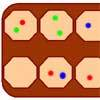Wari

Age 14 to 16 Challenge Level:

This is a simple version of an ancient game played all over the world. It is also called Mancala. What tactics will increase your chances of winning?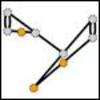Sprouts

Age 7 to 18 Challenge Level:

A game for 2 people. Take turns joining two dots, until your opponent is unable to move.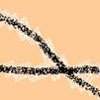Making Tracks

Age 14 to 16 Challenge Level:

A bicycle passes along a path and leaves some tracks. Is it possible to say which track was made by the front wheel and which by the back wheel?Changing Places

Age 14 to 16 Challenge Level:

Place a red counter in the top left corner of a 4x4 array, which is covered by 14 other smaller counters, leaving a gap in the bottom right hand corner (HOME). What is the smallest number of moves. . . .Cubic Net

Age 14 to 18 Challenge Level:

This is an interactive net of a Rubik's cube. Twists of the 3D cube become mixes of the squares on the 2D net. Have a play and see how many scrambles you can undo!Instant Insanity

Age 11 to 18 Challenge Level:

Given the nets of 4 cubes with the faces coloured in 4 colours, build a tower so that on each vertical wall no colour is repeated, that is all 4 colours appear.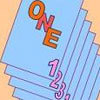One Out One Under

Age 14 to 16 Challenge Level:

Imagine a stack of numbered cards with one on top. Discard the top, put the next card to the bottom and repeat continuously. Can you predict the last card?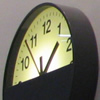Clocking Off

Age 7 to 16 Challenge Level:

I found these clocks in the Arts Centre at the University of Warwick intriguing - do they really need four clocks and what times would be ambiguous with only two or three of them?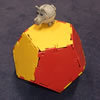Platonic Planet

Age 14 to 16 Challenge Level:

Glarsynost lives on a planet whose shape is that of a perfect regular dodecahedron. Can you describe the shortest journey she can make to ensure that she will see every part of the planet?Speeding Boats

Age 14 to 16 Challenge Level:

Two boats travel up and down a lake. Can you picture where they will cross if you know how fast each boat is travelling?Just Rolling Round

Age 14 to 16 Challenge Level:

P is a point on the circumference of a circle radius r which rolls, without slipping, inside a circle of radius 2r. What is the locus of P?Triangles in the Middle

Age 11 to 18 Challenge Level:

This task depends on groups working collaboratively, discussing and reasoning to agree a final product.Square It

Age 11 to 16 Challenge Level:

Players take it in turns to choose a dot on the grid. The winner is the first to have four dots that can be joined to form a square.Fermat's Poser

Age 14 to 16 Challenge Level:

Find the point whose sum of distances from the vertices (corners) of a given triangle is a minimum.How Many Dice?

Age 11 to 14 Challenge Level:

A standard die has the numbers 1, 2 and 3 are opposite 6, 5 and 4 respectively so that opposite faces add to 7? If you make standard dice by writing 1, 2, 3, 4, 5, 6 on blank cubes you will find. . . .Jam

Age 14 to 16 Challenge Level:

To avoid losing think of another very well known game where the patterns of play are similar.The Perforated Cube

Age 14 to 16 Challenge Level:

A cube is made from smaller cubes, 5 by 5 by 5, then some of those cubes are removed. Can you make the specified shapes, and what is the most and least number of cubes required ?Ding Dong Bell

Age 11 to 18

The reader is invited to investigate changes (or permutations) in the ringing of church bells, illustrated by braid diagrams showing the order in which the bells are rung.Painting Cubes

Age 11 to 14 Challenge Level:

Imagine you have six different colours of paint. You paint a cube using a different colour for each of the six faces. How many different cubes can be painted using the same set of six colours?Icosian Game

Age 11 to 14 Challenge Level:

This problem is about investigating whether it is possible to start at one vertex of a platonic solid and visit every other vertex once only returning to the vertex you started at.Inside Out

Age 14 to 16 Challenge Level:

There are 27 small cubes in a 3 x 3 x 3 cube, 54 faces being visible at any one time. Is it possible to reorganise these cubes so that by dipping the large cube into a pot of paint three times you. . . .Dice, Routes and Pathways

Age 5 to 14

This article for teachers discusses examples of problems in which there is no obvious method but in which children can be encouraged to think deeply about the context and extend their ability to. . . .Sliding Puzzle

Age 11 to 16 Challenge Level:

The aim of the game is to slide the green square from the top right hand corner to the bottom left hand corner in the least number of moves.Königsberg

Age 11 to 14 Challenge Level:

Can you cross each of the seven bridges that join the north and south of the river to the two islands, once and once only, without retracing your steps?Building Tetrahedra

Age 14 to 16 Challenge Level:

Can you make a tetrahedron whose faces all have the same perimeter?Jam

Age 14 to 16 Challenge Level:

A game for 2 players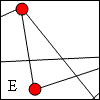Travelling Salesman

Age 11 to 14 Challenge Level:

A Hamiltonian circuit is a continuous path in a graph that passes through each of the vertices exactly once and returns to the start. How many Hamiltonian circuits can you find in these graphs?Tetrahedra Tester

Age 11 to 14 Challenge Level:

An irregular tetrahedron is composed of four different triangles. Can such a tetrahedron be constructed where the side lengths are 4, 5, 6, 7, 8 and 9 units of length?Picturing Square Numbers

Age 11 to 14 Challenge Level:

Square numbers can be represented as the sum of consecutive odd numbers. What is the sum of 1 + 3 + ..... + 149 + 151 + 153?Picturing Triangular Numbers

Age 11 to 14 Challenge Level:

Triangular numbers can be represented by a triangular array of squares. What do you notice about the sum of identical triangle numbers?Cube Paths

Age 11 to 14 Challenge Level:

Given a 2 by 2 by 2 skeletal cube with one route `down' the cube. How many routes are there from A to B?Paving Paths

Age 11 to 14 Challenge Level:

How many different ways can I lay 10 paving slabs, each 2 foot by 1 foot, to make a path 2 foot wide and 10 foot long from my back door into my garden, without cutting any of the paving slabs?Tourism

Age 11 to 14 Challenge Level:

If you can copy a network without lifting your pen off the paper and without drawing any line twice, then it is traversable. Decide which of these diagrams are traversable.Khun Phaen Escapes to Freedom

Age 11 to 14 Challenge Level:

Slide the pieces to move Khun Phaen past all the guards into the position on the right from which he can escape to freedom.Cutting a Cube

Age 11 to 14 Challenge Level:

A half-cube is cut into two pieces by a plane through the long diagonal and at right angles to it. Can you draw a net of these pieces? Are they identical?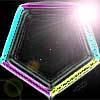Penta Colour

Age 14 to 16 Challenge Level:

In how many different ways can I colour the five edges of a pentagon red, blue and green so that no two adjacent edges are the same colour?Something in Common

Age 14 to 16 Challenge Level:

A square of area 3 square units cannot be drawn on a 2D grid so that each of its vertices have integer coordinates, but can it be drawn on a 3D grid? Investigate squares that can be drawn.3D Treasure Hunt

Age 14 to 18 Challenge Level:

Some treasure has been hidden in a three-dimensional grid! Can you work out a strategy to find it as efficiently as possible?Prime Magic

Age 7 to 16 Challenge Level:

Place the numbers 1, 2, 3,..., 9 one on each square of a 3 by 3 grid so that all the rows and columns add up to a prime number. How many different solutions can you find?Just Opposite

Age 14 to 16 Challenge Level:

A and C are the opposite vertices of a square ABCD, and have coordinates (a,b) and (c,d), respectively. What are the coordinates of the vertices B and D? What is the area of the square?Cogs

Age 11 to 14 Challenge Level:

A and B are two interlocking cogwheels having p teeth and q teeth respectively. One tooth on B is painted red. Find the values of p and q for which the red tooth on B contacts every gap on the. . . .Euromaths

Age 11 to 14 Challenge Level:

How many ways can you write the word EUROMATHS by starting at the top left hand corner and taking the next letter by stepping one step down or one step to the right in a 5x5 array?Auditorium Steps

Age 7 to 14 Challenge Level:

What is the shape of wrapping paper that you would need to completely wrap this model?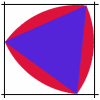Rolling Triangle

Age 11 to 14 Challenge Level:

The triangle ABC is equilateral. The arc AB has centre C, the arc BC has centre A and the arc CA has centre B. Explain how and why this shape can roll along between two parallel tracks.Weighty Problem

Age 11 to 14 Challenge Level:

The diagram shows a very heavy kitchen cabinet. It cannot be lifted but it can be pivoted around a corner. The task is to move it, without sliding, in a series of turns about the corners so that it. . . .Tied Up

Age 14 to 16 Short Challenge Level:

How much of the field can the animals graze?Route to Infinity

Age 11 to 14 Challenge Level:

Can you describe this route to infinity? Where will the arrows take you next?Age 11 to 14 Challenge Level:

Four rods, two of length a and two of length b, are linked to form a kite. The linkage is moveable so that the angles change. What is the maximum area of the kite?Trice

Age 11 to 14 Challenge Level:

ABCDEFGH is a 3 by 3 by 3 cube. Point P is 1/3 along AB (that is AP : PB = 1 : 2), point Q is 1/3 along GH and point R is 1/3 along ED. What is the area of the triangle PQR?Take Ten

Age 11 to 14 Challenge Level:

Is it possible to remove ten unit cubes from a 3 by 3 by 3 cube so that the surface area of the remaining solid is the same as the surface area of the original?